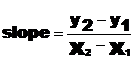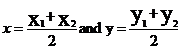Lab 2 - Whose Line Is It Anyway?

Write a complete C++ program that declares  and assigns four values that represent two points on a line.  The program calculates and displays the slope and the midpoint of that line.

Given two points (x1, y1) and (x2, y2) the formula for the slope of a line is:The formula for the midpoint of a line is:

midpoint = (x, y) whereBE CAREFUL.  Sometimes the result is a floating point number.  You need to be conscious of integer/float division.

Test your program with the following three sets of points and one set of your own choosing.

 Line Point1 Point2 A (4,6) (6, 18) B (-10, 8) (14, 4) C (3.1, -6) (20, 16)

Your output should display the original two points, the slope and the midpoint in a clear organized manner.

The only change you have to make to run all inputs is the values of your assignment statements.  In other words your cout statements should use the variable names and not the values given.

Sample Output

The first point's coordinate is: (4,7)

The second point's coordinate is: (6,18)

The slope of the line is: 5.5

The midpoint is: (5,12.5)

IMPORTANT - Place the steps for the software development procedure in a comment at the top of your program.

HAND IN - Your program along with the 4 sets of output produced.